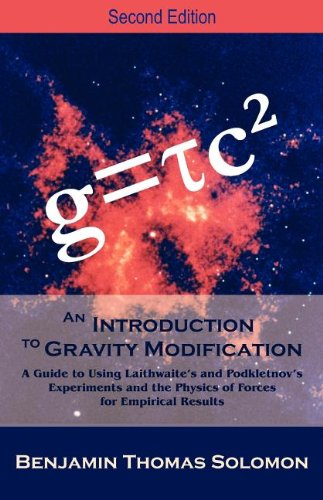## g=τc^2What does it mean to say that gravitational acceleration is  neither a function of the mass of the gravitational source nor a function of gravitational waves?

It means, in my lay person’s view, that anti-gravity technology is one short paradigm shift away. Of course, physicists make no such careless overstatements. But they do speak of gravity modification, and g=τc2 means it is possible to infer gravity modifying effects using non-inertia Ni field.

That is the subject of Benjamin T. Solomon’s 2012 book, An Introduction to Gravity Modification: A Guide to Using Laithwaite’s and Podkletnov’s Experiments and the Physics of Forces for Empirical Results. Eric Roberts Laithwaite (June 14, 1921 – November 27, 1997) was an electrical engineer known as the “Father of Maglev” for his development of the linear induction motor and maglev rail system. Eugene Podkletnov is a ceramics engineer known for experiments first reported in the 1990s demonstrating gravity shielding with the use of superconducting rotating disks.

According to ScienceDirect, “Solomon used process models to introduce the concept of non-inertia Ni fields and derive the massless gravitational acceleration formula g=τc2… Solomon had also shown that many photon experimental results could be modeled without the use of quantum theory. This would imply that neither a quantum nor a relativistic type theory would be indispensable to formulating a theory on gravity modification.”

From the book blurb:

“The analytical formulation and numerical integration has led to the discovery of a new formula for gravitational acceleration, g = τc2, that is neither a function of the mass of the gravitational source nor a function of gravitational waves; where τ is a function of the time dilation present in the local gravitational field. This formula has been tested and verified to be correct in the gravitational fields of the nine planetary bodies in our Solar System and the Sun: mechanical acceleration, and electromagnetic fields. Thus leading to the inference that g = τc2 is the generic formula for all non-nuclear force fields. The true power of this definition of gravitational acceleration lies in the fact that it now lends itself to a portable technology, as mass is no longer required to derive acceleration. This new relationship for acceleration describes how an electron moving in a magnetic field causes a force on the electron and explains why the electron velocity, magnetic field, and resulting force relationship is orthogonal.”

This is pretty fascinating stuff, although I wish right now I was back in school signing up for a few more physics classes. But don’t panic. “The mathematics is easier than that taught in theoretical physics and therefore accessible to a wider audience such as these engineers and technologists.”

Solomon’s treatise on a new approach to gravity commands a moderately hefty price–\$49.10 USD on Amazon as this is written. But compared to physics textbooks at any modern university, the 532-page work seems a steal.

This entry was posted in Science and tagged , . Bookmark the permalink.